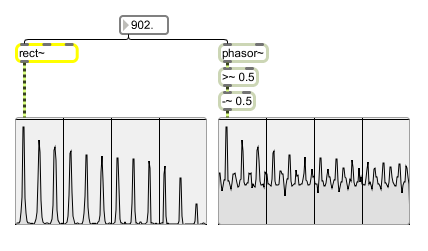rect~

Antialiased rectangular (pulse) oscillator

Description

rect~ generates a rectangle wave whose component frequencies are resistant to aliasing.

Arguments

Name Type Opt Description
frequency and pulse-width int or float opt First argument sets the initial frequency of the oscillator. The default is 0. Second argument sets the pulse width. The default is 0.5.

Messages

 int frequency [int] In left inlet: Sets the frequency of the oscillator. In middle inlet: Sets the pulse width of the oscillator. Signal is wrapped into the range 0-1. A value of 0.5 will produce a rectangular wave that spends equal amounts of time on the positive and negative edges of its cycle. float frequency [float] In left inlet: Sets the frequency of the oscillator. In middle inlet: Sets the pulse width of the oscillator. Signal is wrapped into the range 0-1. A value of 0.5 will produce a rectangular wave that spends equal amounts of time on the positive and negative edges of its cycle. signal In left inlet: Sets the frequency of the oscillator. In middle inlet: Sets the pulse width of the oscillator. Signal is wrapped into the range 0-1. A value of 0.5 will produce a rectangular wave that spends equal amounts of time on the positive and negative edges of its cycle. In right inlet: (optional) A sync signal. When the control signal crosses from below 0.5 to above 0.5, the oscillator resets itself. A phasor~ object works well for this purpose. The classic use is to set this control signal to your fundamental frequency and "sweep" the left frequency input in a range somewhere several octaves higher than the fundamental. synctrig sync-value (0 through 1) [float] The word synctrig followed by a floating-point number changes the value at which the object resets itself (i.e., when the input signal crosses from below the specified number to being above it, rect~ will reset itself).

Output

signal: An antialiased rectangular waveform. An ideal, straight-line rectangular wave generated in a computer contains aliased frequencies that can sound irritating. rect~ produces a nice, analog-esque output waveform.

ExamplesSpectral comparison of rect~ and an ideal rectangular wave driven by a phasor~